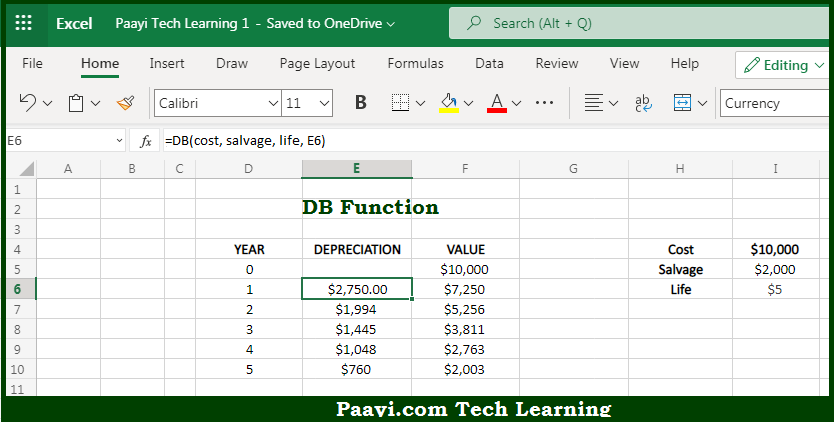# Learn How to Use Microsoft Excel DB Function

Written by | 0 Comments | 560 Views

In this article, you will learn how to use the Microsoft Excel DB function and its prime function in Microsoft Excel. You will also get to know the Microsoft Excel DB function return value and syntax with the help of some examples.

Microsoft Excel DB Function

The main purpose of the Microsoft Excel DB function is to get the depreciation - fixed - declining balance. That implies, with the help of the DB function you can able to return the depreciation of an asset for a specified period using the fixed-declining balance method. The calculation is based on initial asset cost, salvage value, the number of periods over which the asset is depreciated, and, optionally, the number of months in the first year. So, with the help of the DB function, you can able to the depreciation - fixed - declining balance.

Return Value of DB Function

The return value will be the depreciation in the given period.

Syntax of DB Function

=DB(cost, salvage, life, period, [month])

Where the arguments:

• cost: This is the initial cost of the asset.
• salvage: This is the asset value at the end of depreciation.
• life: This is the period over which the asset is depreciated.
• period: This is the period for which the depreciation is calculated.
• month: These are a number of months in the first year. Defaults to 12 (optional).

How to Use Microsoft Excel DB Function?So we know that Microsoft Excel DB function you can able to get the depreciation - fixed - declining balance. That implies, with the help of the DB function you can able to return the depreciation of an asset for a specified period using the fixed-declining balance method. The calculation is based on initial asset cost, salvage value, the number of periods over which the asset is depreciated, and, optionally, the number of months in the first year. So, with the help of the DB function, you can able to the depreciation - fixed - declining balance.